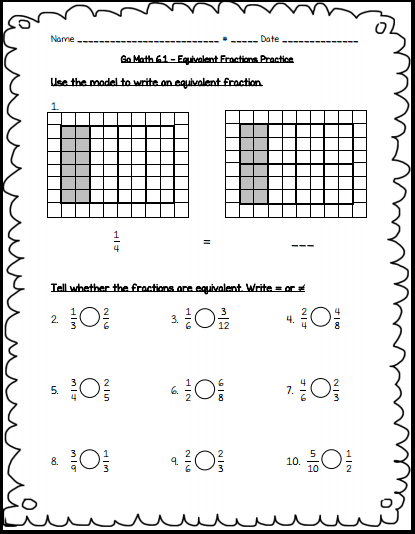## Grade 6 Maths Fractions Multiple Choice Questions (MCQs)

1. Write the fraction representing the shaded region in the below left figure.
(a)$\frac{1}{8}$
(b)$\frac{4}{8}$
(c)$\frac{5}{8}$
(d)$\frac{3}{8}$2. Write the fraction representing the shaded region in the above sided right figure.
(a)$\frac{1}{8}$
(b)$\frac{4}{8}$
(c)$\frac{5}{8}$
(d)$\frac{3}{8}$

3. Fill in the boxes with the correct symbol:$\frac{1}{2} \square \frac{3}{2}$
(a) >
(b) <
(c) =
(d) none of these

4. Fill in the boxes with the correct symbol:$\frac{3}{4} \square \frac{2}{4}$
(a) >
(b) <
(c) =
(d) none of these

5. Fill in the boxes with the correct symbol:$\frac{5}{8} \square \frac{7}{8}$
(a) >
(b) <
(c) =
(d) none of these

6. Fill in the boxes with the correct symbol:$\frac{5}{5} \square \frac{7}{7}$
(a) >
(b) <
(c) =
(d) none of these

7. What fraction of a day is 8 hours?
(a)$\frac{1}{8}$
(b)$\frac{8}{1}$
(c)$\frac{3}{1}$
(d)$\frac{1}{3}$

8. What fraction of an hour is 45 minutes?
(a)$\frac{4}{3}$
(b)$\frac{3}{4}$
(c)$\frac{1}{2}$
(d)$\frac{4}{5}$

9. The equivalent fraction of$\frac{3}{5}$ with denominator 20 is:
(a)$\frac{12}{20}$
(b)$\frac{20}{12}$
(c)$\frac{10}{20}$
(d)$\frac{15}{20}$

10. The equivalent fraction of y with numerator 9 is:
(a)$\frac{15}{9}$
(b)$\frac{9}{11}$
(c)$\frac{9}{15}$
(d)$\frac{9}{5}$

11. The simplest form of$\frac{48}{60}$ is:
(a)$\frac{5}{4}$
(b)$\frac{4}{5}$
(c)$\frac{8}{10}$
(d)$\frac{12}{15}$

12. Which one of the following is a proper fraction?
(a)$\frac{5}{6}$
(b)$\frac{7}{3}$
(c)$\frac{4}{3}$
(d)$\frac{8}{5}$

13. Which one of the following is an improper fraction?
(a)$\frac{7}{8}$
(b)$\frac{8}{3}$
(c)$\frac{3}{4}$
(d)$\frac{9}{11}$

14. A proper fraction with denominator 7 is:
(a)$\frac{8}{7}$
(b)$\frac{4}{7}$
(c)$\frac{9}{7}$
(d)$\frac{11}{7}$

15. An improper fraction with denominator 9 is:
(a)$\frac{2}{9}$
(b)$\frac{7}{9}$
(c)$\frac{11}{9}$
(d)$\frac{5}{9}$

16.$\frac{20}{3}$ can be written in mixed fraction as:
(a) 3$\frac{6}{2}$
(b) 6$\frac{2}{3}$
(c) 2$\frac{6}{3}$
(d) 5$\frac{5}{3}$

17. 6$\frac{2}{3}$ can be written in improper fraction as:
(a)$\frac{3}{20}$
(b)$\frac{15}{3}$
(c)$\frac{20}{3}$
(d)$\frac{3}{15}$

18. Which of the following can be written in the box$\frac{2}{7}=\frac{8}{\square}$?
(a) 16
(b) 13
(c) 28
(d) 35

19. Which of the following can be written in the box$\frac{3}{5}=\frac{\square}{20}$?
(a) 18
(b) 12
(c) 60
(d) 15

20. The next equivalent fraction of the given fraction:$\frac{1}{2}, \frac{2}{4}, \frac{3}{6}, \frac{4}{8}, \ldots \ldots . ., \text { is }$
(a)$\frac{7}{14}$
(b)$\frac{6}{12}$
(c)$\frac{10}{5}$
(d)$\frac{5}{10}$

### Class 6 Maths Fractions Fill in the blanks

1. A number representing a part of a ………………. is called a fraction.
2. A fraction with denominator greater than the numerator is called a …………….. fraction.
3. Fractions with the same denominator are called ……………….. fractions.
4. 13$\frac{5}{8}$ is a …………… fraction.
5.$\frac{5}{8}$ and$\frac{3}{8}$ are ………………… proper fractions.
6.$\frac{6}{11}$ and$\frac{6}{13}$ are ……………… proper fractions.
7. The fraction$\frac{17}{34}$ in simplest form is …………….. .
8. The fraction$\frac{6}{15}$ in simplest form is …………… .
9. When$\frac{1}{4}$ is written with denominator as 12, its numerator is ………….. .
10. The value of 1 +$\frac{2}{3}$ is ……………… .

### Class 6 Maths Fractions Very Short Answer Type Questions

1. Add the fraction$\frac{3}{8}$ and$\frac{5}{8}$.
2. Subtract$\frac{11}{4}$ from$\frac{61}{4}$.
3. A girl dyes dresses. She had to dye 30 dresses. She has so far finished 20 dresses. What fraction of dresses has she finished?
4. Compare$\frac{4}{5}$ and$\frac{7}{5}$.
5. Shubham painted$\frac{2}{3}$ of the wall and his sister painted$\frac{1}{3}$ of the wail space. How much did they paint together?
6. Javed was given$\frac{5}{7}$ of a basket of oranges. What fraction of oranges was left in the basket?

### Class 6 Maths Fractions Short Answer Type Questions

1. What fraction of an hour is 40 minutes?
2. Subtract$\frac{81}{3}$ from$\frac{100 }{9}$.
3. A rectangle is divided into certain number of equal parts. If 16 of the parts so formed represent the fraction$\frac{1}{4}$, find the number of parts in which the rectangle has been divided.
4. Grip size of a tennis racquet is 11$\frac{9}{80}$ cm; express the size as an improper fraction.

### Class 6 Maths Fractions Long Answer Type Questions

1. Arya, Abhimanyu and Vivek shared lunch. Arya has brought two sandwiches, one made of vegetable and one of jam. The other two boys forgot to bring their lunch. Arya agreed to share his sandwiches so that each person will have an equal share of each sandwich.
(a) How can Arya divide his sandwiches so that each person has an equal share?
(b) What part of a sandwich will each boy receive?
2. Find the equivalent fraction of$\frac{3}{5}$ having:
(a) denominator 20
(b) numerator 9
(c) denominator 30
(b) numerator 27
3. Ramesh had 20 pencils, Sheelu had 50 pencils and Jamaal had 80 pencils. After 4 months, Ramesh used up 10 pencils, Sheelu used up 25 pencils and Jamaal used up 40 pencils. What fraction did each use up? Check if each has used up an equal fraction of her/his pencils?Maths fractions worksheets for grade 6